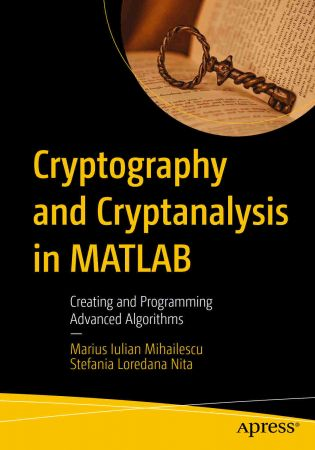# Cryptography and Cryptanalysis in MATLAB Creating and Programming Advanced Algorithms110

#### TOP

##### Alpha and Omega
Member
AccessEnglish | 2021 | ISBN: 1484273338 | 201 pages | True (PDF, EPUB) | 11.79 MB
Master the essentials of cryptography and cryptanalysis and learn how to put them to practical use. Each chapter of this book starts with an introduction to the concepts on which cryptographic algorithms are based and how they are used in practice, providing fully working examples for each of the algorithms presented. Implementation sections will guide you through the entire process of writing your own applications and programs using MATLAB.

Cryptography and Cryptanalysis in MATLAB will serve as your definitive go-to cryptography reference, whether you are a student, professional developer, or researcher, showing how a multitude of cryptographic challenges can be overcome using the powerful tools of MATLAB.
What You Will Learn

Discover MATLAB's cryptography functions
Work with conversion mechanisms in MATLAB
Implement cryptographic algorithms using arithmetic operations
Understand the classical, simple cryptosystems that form the basis of modern cryptography
Develop fully working solutions (encryption/decryption operations)
Study pseudo-random generators and their real-life implementations
Utilize hash functions by way of practical examples
Implement solutions to defend against practical cryptanalysis methods and attacks
Understand asymmetric and symmetric encryption systems and how to use them
Leverage visual cryptography, steganography, and chaos-based cryptography
Who This Book Is For

Those who are new to cryptography/analysis. Some prior exposure to MATLAB recommended.Replies
0
Views
36
TOPReplies
0
Views
49
TOPReplies
0
Views
35
TOPReplies
0
Views
59
TOPReplies
0
Views
24
TOP